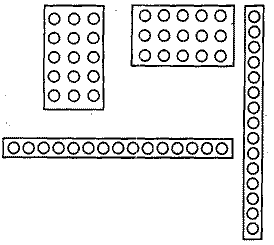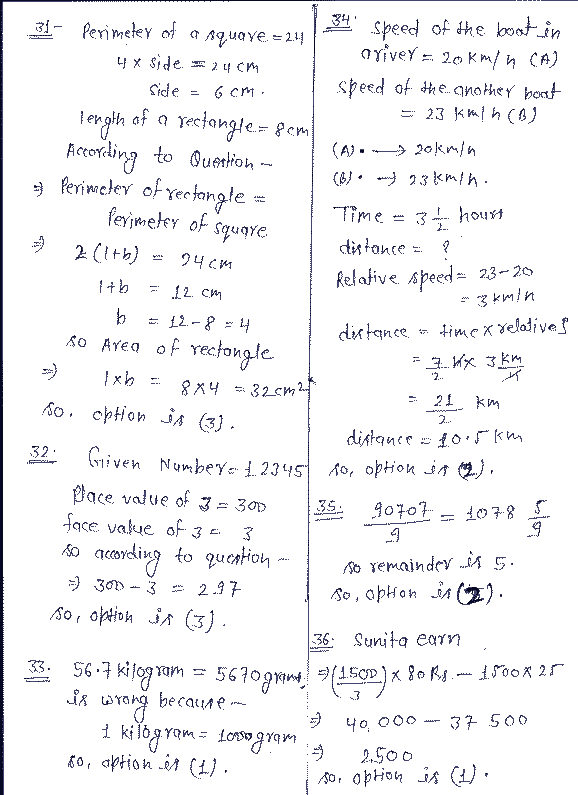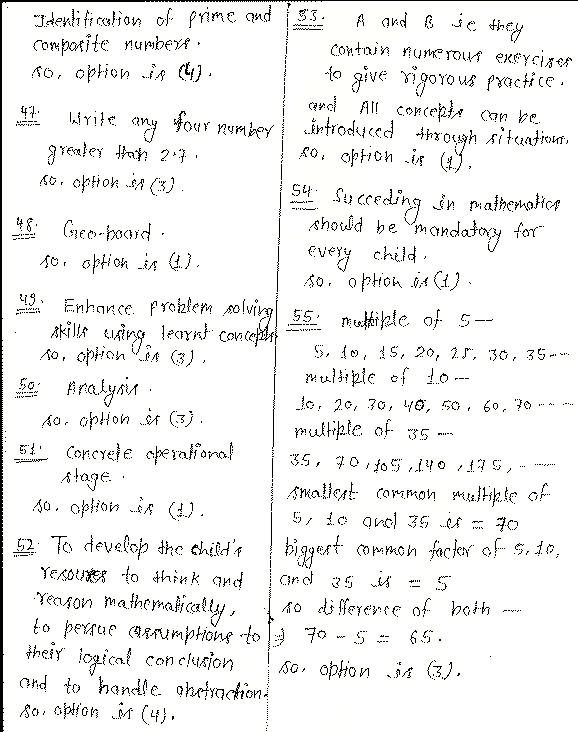# CBSE 2021 CTET Solved Question paper Paper 2 Answer Key

question paper CTET July 2022 maths social science child development and pedagogy English Hindi with answer key and question paper solution provided by upjob.in official answer sheet and question paper of previous years CTET examination download PDF file

CBSE Solved Question paper Upper Primary level Paper 2 | Mathematics question paper solution for paper 2  | Model Sample question paper for UPTET / Teacher Eligibility Test Upper primary level junior Level Sample question paper download Free of cost CTET  February 2022

Upper Primary Level UPS Question Paper CTET Examination Solved question Paper
MATHEMATICS MODAL PAPER FOR  CTET / UPTET

31. Perimeter of a square is 24 cm and length of a rectangle is 8 cm., If the perimeters of the square and the rectangle are equal, then the area (in square cm) of the rectangle is
(1) 16        (2) 24        (3) 32        (4) 64

32. The difference of the place value and the face value of the number 3 in 12345 is
(1) 0        (2) 295        (3) 297        (4) 305

33. Which one of the following is not correct?
(1) 56.7 kilogram = 5670 grams
(2) A cube has six faces.
(3) One millimeter =O.1 cm
(4) 0.10 is same as 0.1

34. The speed of a boat in a river is 20 km per hour and the speed of another boat is 23 km per hour. They travel in the same direction from the same place at the same time. The distance between the boats after three and half hours is
(1) 10 km        (2) 10.5 km        (3) 11 km        (4) 11.5 km

35. When 90707 is divided by 9, the remainder is
(1) 3            (2) 5            (3) 6            (4) 7

36 .When a fresh fish is dried it becomes 1/3 of its weight. Sunita buys 1500 kg fresh fish for 25 per kg and sell them, when dried, for 80 per kg. How much does she earn?
(1) Rs 2,5O0        (2) Rs 2,700        (3) Rs 3,000        (4) Rs 3,500

37. Look at the following pattern:
(9 -1) ÷ 8 = 1
(98 -2) ÷ 8 =12
(987 -3) ÷ 8 = 123
(9876 -4) ÷ 8 =1234
According to this pattern
(987654 -6)  ÷ 8 =
(1) 12345        (2) 123456        (3) 123465        (4) 123467

38. 750 ml juice is, filled in one bottle and six such bottles are packed in one carton. The number of cartons needed for 450 litres of juice is
(1) 75            (2) 80            (3) 90            (4) 100

39. Internal length, breadth and depth of a (rectangular) box are 4 cm, 3 cm and 2 cm respectively. How many , such boxes are needed to pack 8664 centimetre cubes?
(1) 351            (2) 361            (3) 391            (4) 722

40. “Write the equivalent fraction of  1/3”

The above question asked to students of Class IV refers to
(1) lower-level demand task as it requires procedural skills only.
(2) lower-level demand task as it is based on memorization only.
(3) higher-level demand task as it is based on procedure with connection.
(4) higher-level demand task as it is based on procedure without connection.

41. Students often make a mistake in comparing the decimal numbers. For example 0.50 is larger than 0.5. The most probable reason for this error is
(1) lack of practice of these types of questions in the class.
(2) lack of concrete experience of representation of decimal number on number line.
(3) careless attempt by the students.
(4) misconception regarding the significance of zero in ordering decimal.

42. A teacher prompts the students to prepare. Mathematical journal with the theme “Application of Mathematics in Daily life”. This activity is
(1) to test the students understanding of Mathematical concepts.
(2) to provide opportunity to students share their ideas and knowledge.
(3) to help students to sense of Mathematics.
(4) to help students to connect Mathematical concepts arid its applications and to share their knowledge and ideas

43. According to Van Hiele level of geometric thought, the five levels are — visualization, analysis, informal deduction, formal deduction and rigour. Some polygons are given to a child of Class III for sorting..
He classified the polygons on the basis of the number of sides. This child is at _________ level Geometrical thought of Van-Hiele(1) Visualization        (2) Analysis
(3) Informal deduction    (4) Formal deduction

44. A child displays difficulty in differentiating between numbers, operations and  symbols, two clock hands, different coins etc. This implies that the specific barrier affecting his. learning is
(1) poor verbal, visual, auditory and working memory.
(2) poor visual processing ability i.e. visual discrimination, spatial organization and visual coordination.
(3) poor language processing ability i.e. expression, vocabulary and auditory processing.
(4) poor motor skills, reading and writing skills.

45. NCF 2005 emphasises on Constructivist Approach of learning as it focuses on
(1) memorization of definitions and formulae.
(2) submission of regular homework
(3) active participation of learner through engaging activities.
(4) effective lecture and instructions by teacher.46. Following are array diagram using bindis to represent 15.
The way of representing 15 or any other number in the above manner can be used to teach concept of

(I) area and commutative property
(2) commutative property of multiplication, identification of prime and composite    numbers, area of rectangle
(3) representation of a number as product of two numbers, commutative property of multiplication, multiplicative identity, identification of prime and composite numbers, area of rectangle using units quantity
(4) representation of a number as product of two numbers, commutative property of multiplication, multiplication identity, identification of prime and composite numbers.

47. Which of the following questions is open-ended?
(1) Write the numbers 25, 71, 19, 9, 8, 17, 85 in ascending order.
(2) Which is more?
1/3 or 7/5
(3) Write any four  number  greater than 2.7.
(4) What is 7 more than  2/7  ?

48. The most appropriate tool to expose the students of class II to plane figures, its   vertices and edges is
(1) Geo-Board                (2) Nets of 3D solids
(3) Cubes                (4) Black-board surface

49. Following is a problem from text book of class V:
“There are 4 poles of measure 105 cm, 215 cm, 150 cm and 235 cm respectively. If they have to be cut into pieces of equal length, what is the maximum length of each piece ?“
(1) test knowledge of factors and mu1tiple
(2) check the skill of finding HCF
(3) enhance problem solving skills using learnt concepts
(4) give practice of word problems. based on HCF and LCM

50. Following is a problem from text book of class III:
“Which mathematical operation will be used to solve the following problem?
A milkman sold 1410 litres of milk in 10 days. How many litres of milk did he sell in a day ?“
Which competence of Bloom’s cognitive domain is referred in the above question?
(1) Knowledge            (2) Comprehension
(3) Analysis                (4) Synthesis

51. Rashid is studying in class V. He can classify various types of triangles in different categories but has difficulty in understanding the abstract proof for the sum of three angles in a triangle to be always 180. According to Piaget Cognitive Theory Rashid is
at
(1) Concrete operational stage
(2) Formal operational  stage
(3) Sensorimotor stage
(4) Pre-operational stage

52. According to NCF 2005

“Developing children ‘s abilities for mathematization is the main goal of mathematics education. The narrow aim of school mathematics is lo develop ‘useful’ capabilities.”
Here mathematization refers to develop child’s abilities

(1) In performing all number operations efficiently including of finding square root and cube root.
(2) To formulate Theorems of Geometry and their proofs independently.
(3) To translate word problems into linear equations.
(4) To develop the child’s resources to think and reason mathematically, to pursue
assumptions to their logical conclusion and to handle abstraction.

53. The highlights of a good textbook are that
A. They contain numerous exercises to give rigorous practice.
B. All concepts can be introduced through situations.
C. Only solved examples are included.
D. They must be thick and heavy.
(1) A and B            (2) C and D
(3) A añd C            (4) B and D

54. NCF 2005 emphasises that
(1) Succeeding in Mathematics should be mandatory for every child.
(2) Students should be tested first for their logico - mathematical ability.
(3) Maths curriculum shall be separate for low achievers.
(4) Maths shall be taught to selective students.

55. The difference between the smallest common multiple and biggest common factor of 5, 10 and 35 is
(1) 30            (2) 35            (3) 65                (4) 75

56. The number of factors of 105 is
(1) 3            (2) 4            (3) 6                (4) 8

57. If the time now is 2.17 P.M., what will be the time 11 hours and 59 minutes from now?
(1) 11.57 A.M.        (2) 09. 59  A.M.
(3) 02.16 A.M.        (4) 02.17 A.M.

58. Number of degrees in three and one half right angles is
(1) 285            (2) 295            (3) 305            (4) 315

59. 11 ones + 11. tens + 11 hundreds equals
(1) 144        (2) 1221            (3) 12321        (4) 111111

60. The sum of five hundred nine and three thousand twenty eight is
(1) 3537    (2) 3087            (3) 837            (4) 387

Upper Primary level Junior Level  JRT  CTET Math Exam Question paper SolutionFor more news related to this, you must join our Telegram channel UPJOBIN so that you can reach the updates first.
Upper primary level  CTET exam official answer key
 Que Ans Que Ans Que Ans 31 3 41 4 51 1 32 3 42 4 52 4 33 1 43 1 53 1 34 2 44 2 54 1 35 2 45 3 55 3 36 1 46 4 56 4 37 2 47 3 57 3 38 4 48 1 58 4 39 2 49 3 59 2 40 1 50 3 60 1